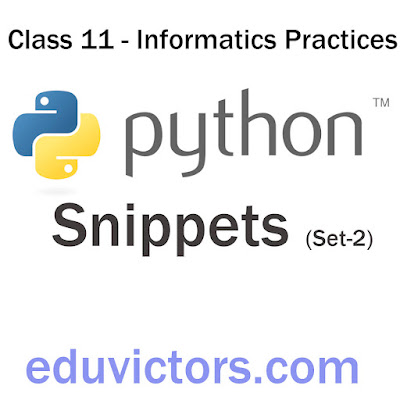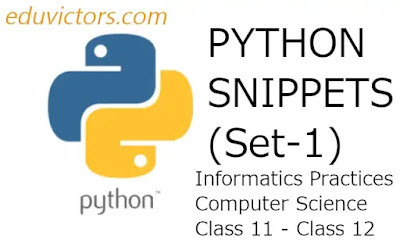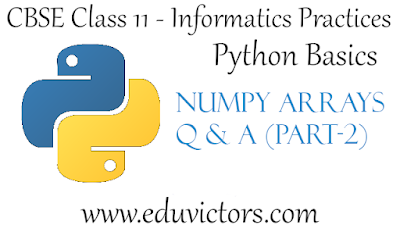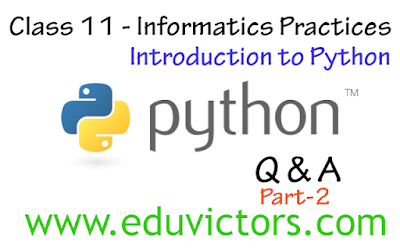## Pages

Showing posts with label class11-Python. Show all posts
Showing posts with label class11-Python. Show all posts

# Class 11 - Informatics Practices - Python Snippets (Set-2)

Write Python Programs for the following problem statements:

1. Write a program in python to input marks in 5 subjects from the user and display the average marks.

2. Write a program in python to read details like name, class, age of a student and then print the details firstly in same line and then in separate lines.

3. Write a program in python to input a number and print its first five multiples.

4. Write a program to read a number n and print n, n² , n³ and n⁴

5. Write a program that generates the following output: 5 @ 10 @ 9# Python Snippets For Class 11 and Class 12

List of Python programs for students studying Informatics Practices and Computer Science.

Program 1: Write a program in python to accept a string and display it in reverse order.

`# write a program in python to accept a string and display it in a reverse order.str1=input("Enter a string: ")print("Reverse string is: ",str1[::-1])`

Output:## Numpy Arrays (Part-1) - Question and Answers

CBSE Class 11 - Informatics Practices - Python Basics

Q1: The following statement has an error. Write the correct statement.
>>> import numpy as np
>>> a = np.array(1,2,3,4)

Answer: The second statement should be a = np.array([1,2,3,4]) to create an ndarray.

Q2: What is the output of the following program?

import numpy as np
list1 = [1,2,3,4,5]
np1 = np.array(list1)
print(type(np))
print(type(np1))
print(np1)
print(np1.shape)

<class 'module'>
<class 'numpy.ndarray'>
3
(5,)## Python Basics - Numpy Arrays

CBSE Class 11 - Informatics Practices

Q1: What is NumPy?

1. NumPy stands for Numerical Python.

2. It is the core library for scientific computing in Python.

3. A NumPy array (also called ndarray) is a homogeneous multidimensional array of data objects.

4. A NumPy array is a table of elements (usually numbers), all of the same type, indexed by a tuple of positive integers.

5. Numpy module provides a set of methods and tools for working with these arrays.

6. In Numpy dimensions are called axes. The number of axes is rank.

Q2: What is the command line option to install NumPy module?

Answer: Run the following command at the command prompt.
pip install numpy

Q3: What is one dimensional (1D) array?

Answer: A one-dimensional array or 1D array is a named group of a contiguous set of elements having the same data type. 1D arrays are also called vectors.

Q4: What is a multi-dimensional array?

Answer: A multidimensional array is an array of arrays. Multi-dimensional arrays are also known as matrices. For example, a two-dimensional array (2D array) has two axes i.e. rows and columns.## Python Basics - List Manipulation (Part-2) - Question and Answers

CBSE Class 11 - Informatics Practices

Based on syllabus of Class 11 Informatics Practices, here are important Questions and Answers covered for the chapter List Manipulation.

The questions and answers will familiarise you with basics concepts of Python Lists and provide a framework for understanding the programming concepts.Software development isn’t any one thing, but a myriad of practices that coalesce into software in the end. Python has been a versatile language and is used by companies to write all sorts of applications.

List is an important data structure, part of a core Python. Lists have a variety of uses including data science, statistics and scientific computing. List is considered as a collection of objects and is ordered and changeable(mutable). It allows duplicate members.

Q1: What is the output of the following code?

list =['I','N','D','I','A']
print(list[0:3])
print(list[3:])
print(list[:])

['I', 'N', 'D']
['I', 'A']
['I','N','D','I','A']

Q2: What is the output of the following code? What does the '+' operator' do in the code snippet?## Python Basics - List Manipulation (Part-1)

CBSE Class 11 - Informatics Practices & Computer Science

Q1: What is a list in Python?

Answer: List is a collections of items and each item has its own index value. It is the most versatile datatype available in core Python which can be written as a list of comma-separated values (items) between square brackets.

e.g.
[] # empty list
[1,2,3,4] #list of integers
[2.0, 4.56, 8.91, ] #list of floats
['Sunday', 'Monday', 'Tuesday'] #list of floats
['Nikita', 16, 'Class 11', True, 86.7] # list of mixed values## Python - String Manipulation (Part-2)

CBSE Class 11 - Informatics Practices

Q1: What are membership operators for strings?

in Returns True if a substring exists in the given string otherwise returns false.
e.g. "xy" in "xyz"  #returns True
"ab" in "xyz"  #returns False

not in Returns True is a substring does not exist in the given string;
e.g. "ab" not in "xyz"   #returns True
"xy"  not in "xyz"   #returns False

Q2: What is the output of the following?
str1 = "Hello World!"
str2 = "he"
str3 = "He"
str2 in str1
str3 in str1## Python Basics - Type Conversion and Type Casting

CBSE Class 11 - Informatics Practices

Question 1: What is Type Conversion?

Answer: Type conversion means converting one data type to another data type. Python supports two types of type conversion:

1. Implicity Type Conversion
2. Explicit Type Conversion (also called Type Casting)

Question 2: What is implicit type conversion? Give an example.## Python - String Manipulation (Part-1)

CBSE Class 11 - Informatics Practices

Q1: What is a string in Python?

Answer: String is a sequence of characters, which is enclosed between either single(' ') quotes or double quotes(" "), python treats both single and double quotes same.
e.g.
str1 = "Welcome to the Python World."

Q2: Can a double-quoted string contain single quotes? If yes, give an example.

Example:
str1 = "Nitika's Fashions"
print(str1)

Similarly, A single-quoted string can also contain double quotes:
Example:
str2 = '"Help!", he exclaimed.'
print(str2)## Python Basics  Q & A (Part-2)

CBSE Class 11 - Informatics Practices

Q1: What are the different modes that can be used to test Python Program?

Answer: In Python, programs can be written in two ways namely Interactive mode and Script mode.
Interactive mode or Interpreter mode allows us to write codes in Python command prompt  (  >>> ).
Script mode is used to create and edit python source file with the extension .py

Q2: Write the command to leave python shell or interpreter.

Answer: ^D (Ctrl+D) or quit () is used to leave the interpreter.

Q3: What is the advantage of interpreter mode?

Answer:  Working in interactive mode is convenient for beginners and for testing small pieces of code, as we can test them immediately.